[BACK]
 Intelligent Automation & Soft ComputingDOI:10.32604/iasc.2023.028047Article

Frequency Control Approach and Load Forecasting Assessment for Wind Systems

Department of EEE, Karpagam College of Engineering, Coimbatore, 641032, India
*Corresponding Author: K. Sukanya. Email: sukanya.k@kce.ac.in
Received: 31 January 2022; Accepted: 10 March 2022

Abstract: Frequency deviation has to be controlled in power generation units when there are fluctuations in system frequency. With several renewable energy sources, wind energy forecasting is majorly focused in this work which is a tough task due to its variations and uncontrollable nature. Whenever there is a mismatch between generation and demand, the frequency deviation may arise from the actual frequency 50 Hz (in India). To mitigate the frequency deviation issue, it is necessary to develop an effective technique for better frequency control in wind energy systems. In this work, heuristic Fuzzy Logic Based Controller (FLC) is developed for providing an effective frequency control support by modeling the complex behavior of the system to enhance the load forecasting in wind based hybrid power systems. Frequency control is applied to reduce the frequency deviation due to fluctuations and load prediction information using ANN (Artificial Neural Network) and SVM (Support Vector Machine) learning models. The performance analysis of the proposed method is done with different machine learning based approaches. The forecasting assessment is done over various climates with the aim to decrease the prediction errors and to demote the forecasting accuracy. Simulation results show that the Mean Absolute Percentage Error (MAPE), Root Mean Square Error (RMSE) and Normalized Mean Absolute Error (NMAE) values are scaled down by 41.1%, 9.9% and 23.1% respectively in the proposed method while comparing with existing wavelet and BPN based approach.

Keywords: Load forecasting; wind power prediction; fuzzy logic controller; ANN; SVM; hybrid power systems

1  Introduction

Globally, the demand for environment friendly renewable energy highlights an unprecedented increase in recent years. The widely popular renewable energy sources are solar, wind, geothermal, biomass. Generally the system frequency fluctuates in the system whenever mismatch occurs between power generation and electricity demand. In recent years, there is sharp increase in the production of wind power to meet the rising power demands of industries and households. Since the wind energy is one of the emerging renewable energy sources for many applications, wind power forecasting is highly essential in wind power generation units and hybrid power systems . Load forecasting is crucial for hybrid power systems due to the presence of renewable energy sources such as wind, solar, hydro and tide. Though the long term and medium term forecasting techniques are possible in wind farms, Short-term forecasting is widely used and reliable to monitor and produce day-to-day wind power generation. It focuses on forecasting from immediate short-term of minutes to 48 h to ensure proper regulation, load balancing and scheduling. Short-term forecasting is also required to avoid fluctuating wind power and to ensure grid stability .

Conventionally, an intermittent power output of wind power system is predicted by deterministic forecasts. However, the deterministic forecasts cannot approximate the uncertainty associated with prediction. Ambiguity associated with wind power forecast (WPF) can be expressed in terms of probabilistic forecasts . Recently frequency control techniques have been improved using machine learning and soft computing techniques in hybrid power systems. In this work, a novel intelligent forecasting technique is presented with frequency control and prediction of wind power. The load frequency disturbance generally occurs in power system when there is disparity between power generation and net power demand. For better frequency control in wind energy systems, frequency corrected demand is necessary for Short term forecasting in wind farms.

Recently frequency control techniques have been improved using machine learning and soft computing techniques. Wavelet based decomposition is applied with ANN in our method to minimize the prediction error. To further improve the accuracy with reduced errors, SVM based technique is included in the final stage of our proposed technique. This paper is structured into five sections including Introduction and review of the existing techniques. Section 2 describes the techniques such as wavelet transform, SVM, ANN, Fuzzy logic which is utilized in this work. The proposed method is dealt with frequency control and forecasting in Section 3. Section 4 discusses the obtained numerical results with comparative analysis on errors and accuracy. Section 5 outlines the conclusion and future directions related to the proposed work.

2  Literature Review

Frequency control techniques are highly required for hybrid power systems than conventional power generation and distribution systems due to fluctuations in system frequency. In hybrid power systems with wind power generation, wind power fluctuations lead to power system frequency variation and stability loss. Recently many intelligent frequency controllers are developed by researchers to improve the performance of conventional frequency controllers. Droop control is employed in hybrid power systems for frequency control using droop coefficient. But conventional droop control methods may result in frequency and amplitude variations while maintaining fixed droop coefficient . To overcome the issues in droop control, adaptive droop control methods are deployed where the droop coefficient is adjusted based on the changes in load and active power . Wind energy based hybrid power systems experience severe frequency variation due to the wind speed fluctuations .

Load frequency control (LFC) techniques are developed over the years to maintain the system frequency to the acceptable levels. System frequency fluctuations need to be controlled by appropriate LFC techniques. Hence there is a requirement to develop intelligent LFC techniques especially in power generation through renewable energy sources. System frequency regulation through intelligent control techniques have been discussed and potential solution has been presented . Hybrid intelligent LFC techniques have been developed in recent years by researchers by adopting neural networks, fuzzy logic, genetic algorithm and optimization algorithms [10,11].

In wind farms, a small imbalance between generation and demand results in frequency fluctuations. Moreover, frequency response of the system is largely affected by asynchronous wind energy generators. In order to make them to work like conventional synchronous generators, it is mandatory to provide some form of control mechanisms . Augmented wind farms and battery energy systems have been reported in the work [13,14] to overcome the frequency control issues. Genetic algorithm (GA) based techniques also focused towards the frequency control and correction using optimization problems . Flywheel algorithm based optimization algorithms have been discussed in literature towards frequency control . Though many techniques are available for wind energy systems, some advanced control techniques with reduced computational elements are very much required to overcome various economic constraints. In our frequency control, FLC is utilized with comparator where deviation in frequency and wind speed data’s are given as input.

The wind power with corrected frequency is applied to the wind power forecasting system. Conventional autoregressive moving average (ARMA) models have been used in the past based on statistical signal processing, however the accuracy needs to be improved in many real time scenarios . Artificial intelligence (AI) approaches like fuzzy ARTMAP (FA), back propagation network (BPN), and SVMs are popular choice among researchers in many prediction applications [18,19]. A comparison between hybrid and artificial intelligence models including support vector machines, expert systems, fuzzy logic, regression trees and artificial neural networks is presented in , while the notable time series models of long short-term memory (LSTM) systems, recurrent (RNN) and convolutional neural networks (CNN) combined with different regression techniques are discussed in . Although highly flexible and effective, RNN-based approaches outperform traditional forecasting models in terms of RMSE and MAPE [24,25].

Particle swam optimization (PSO) based NN and self-organized map (SOM) were also used for the numerical weather prediction (NWP). Based on the survey of numerous papers, it is concluded that SVM requires less computations while comparing with other AI based models . Hence SVM is preferred in the final stage of this work for the prediction with reduced errors.

Forecasting of wind power generation is done using various time series prediction and various soft computing approaches for a specific duration. Wind power prediction is performed with frequency control in few approaches in the recent years, but many works are developed without frequency control methods in the literature. Regression models and ANN based forecasting were implemented in the short term forecasting . These analysis are based on the historical observation for few years. Few works focused towards ANN and fuzzy logic for predicting two to five hours ahead. These works are developed without frequency control and it is difficult to maintain frequency stability in hybrid systems .

However, the techniques discussed above are failed to analyse the short term wind power forecasting even though frequency control is carried out. Similarly, the conventional frequency control may leads to stability problems. Thus, to overcome this problem, this work formulated AI based control topology for frequency control and forecasting with advanced machine learning topologies.

3  Proposed Frequency Control Method for Wind Load Forecasting

The proposed frequency control comprises of two stages, the first stage performs the load frequency control. In the next stage, Frequency controlled data is obtained by comparing change in real power and rate of change of frequency. Recently many advanced techniques such as machine learning algorithms and soft computing techniques are designed to improve the frequency control in wind power system. In this paper, frequency control based on fuzzy inference system is proposed for wind farms and storage units. Fig. 1 shows the elements involved to obtain the frequency controlled data. Fuzzy logic controller (FLC) accepts the wind speed and frequency deviation as inputs and provides the change in real power. The frequency control output is produced by comparator using FLC output and rate of change of frequency. Normally flexible de-loading operation and reduction in storage capacity is achieved in load frequency control technique of wind energy system.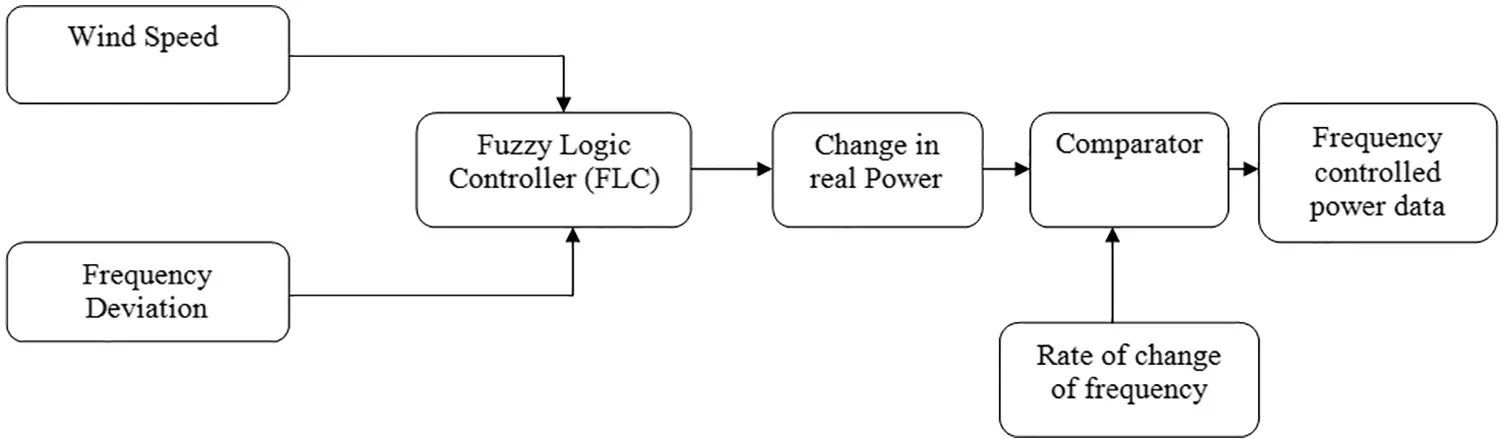Figure 1: Frequency control using fuzzy logic controller

Frequency deviation and wind power data are applied to the fuzzy logic controller. The changes in wind power are considered in the proposed method to improve the performance of demand forecasting. Fig. 2 describes the process involved in the proposed frequency corrected demand forecasting approach based on FLC, ANN and SVM. The frequency corrected wind speed variations are considered for the present and the previous hours. Frequency controlled wind power data series is applied through the wavelet transform to produce decomposed output, whereas frequency controlled wind speed data is given directly to the ANN network. ANN trained data can be recovered using wavelet reconstruction technique. The obtained forecasting errors are applied to SVM classifier to obtain optimized forecasting results.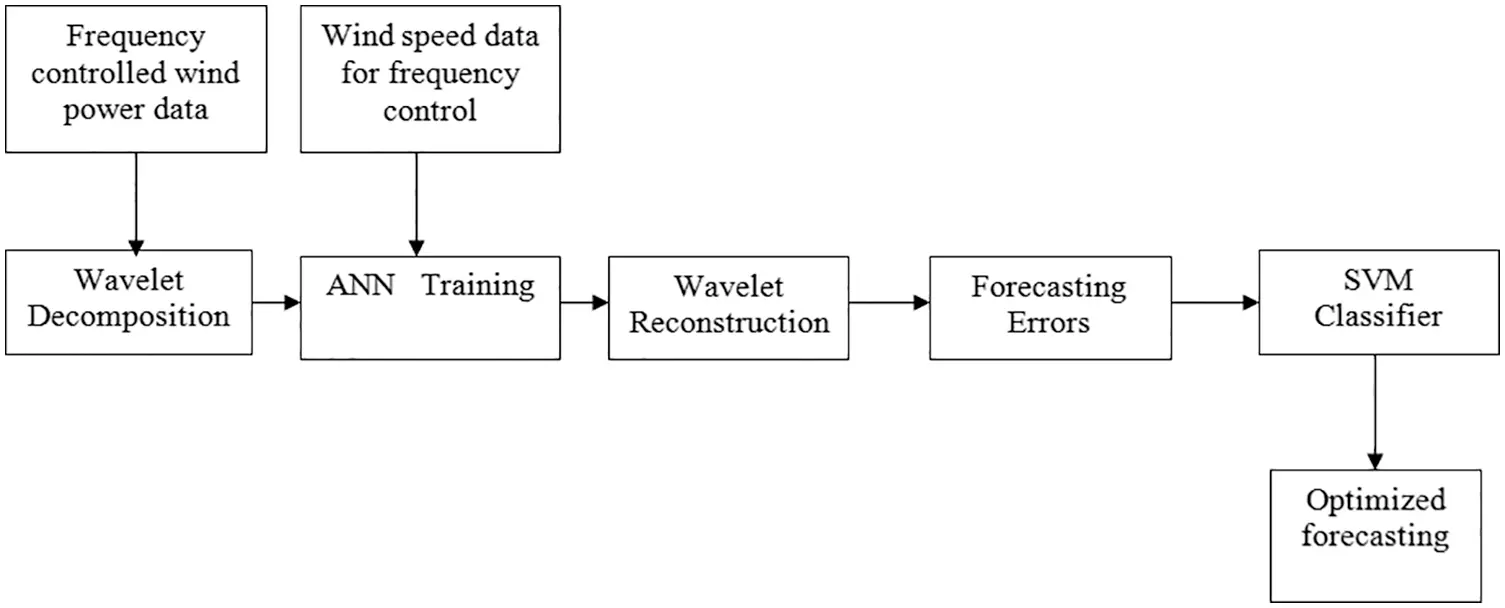Figure 2: Process flow of frequency corrected demand forecasting

The proposed model based on FLC, ANN and SVM provides better forecasting results while comparing with similar existing techniques. To show the superiority of the proposed technique, various error measures like mean square error (MSE), MAPE, RMSE, and NMAE are calculated. Mean square error (MSE) is expressed as

MSEi=Ni=0N(aWPifWPi)2 (1)

where aWP represents actual wind power, fWP denotes forecasted wind power and N is the total number of data points.

MAPE is defined as

MAPE=1Ni=1N|aWPifWPi|aWPiN¯×100% (2)

where aWPiN¯ represents the average true wind power for the Nth hour is obtained by

aWPiN¯=1Ni=1NaWPi (3)

The RMSE is an another important measure which is calculated by

RMSE=1Ni=1N(aWPifWPi)2×100% (4)

The NMAE is calculated by

NMAE=1Ni=1N|aWPifWPi|NPC×100% (5)

where NPC is the name plate capacity of wind farm

4  Simulation Results and Discussion

The proposed load forecasting method is based on wavelet transform, fuzzy logic controller, ANN and SVM. Fuzzy logic controller is developed by considering the wind velocity data and observed frequency variations as the input data. Real wind data has been collected from world’s third largest and India’s largest onshore wind farm located at site Watsun Infrabuild Pvt Ltd, Periyapatti village, Tirupur District. Datasets of wind speed and frequency variations are averaged to obtain hourly data. In that 900 h were used for training (learning set). In that, 180 h were used for cross-validation and 720 h of data have been used for testing the performance. The results presented here are computed on the testing set. Predictions are limited for the next 3 h to improve the forecasting accuracy. The test data set is randomly chosen from July 2020 to April 2021. Tab. 1 shows the samples chosen from the collected data. The model is designed with 36 inputs, 27 neurons and 18 outputs based on daily and weekly forecasting. The computation time is fixed as 1000 epochs.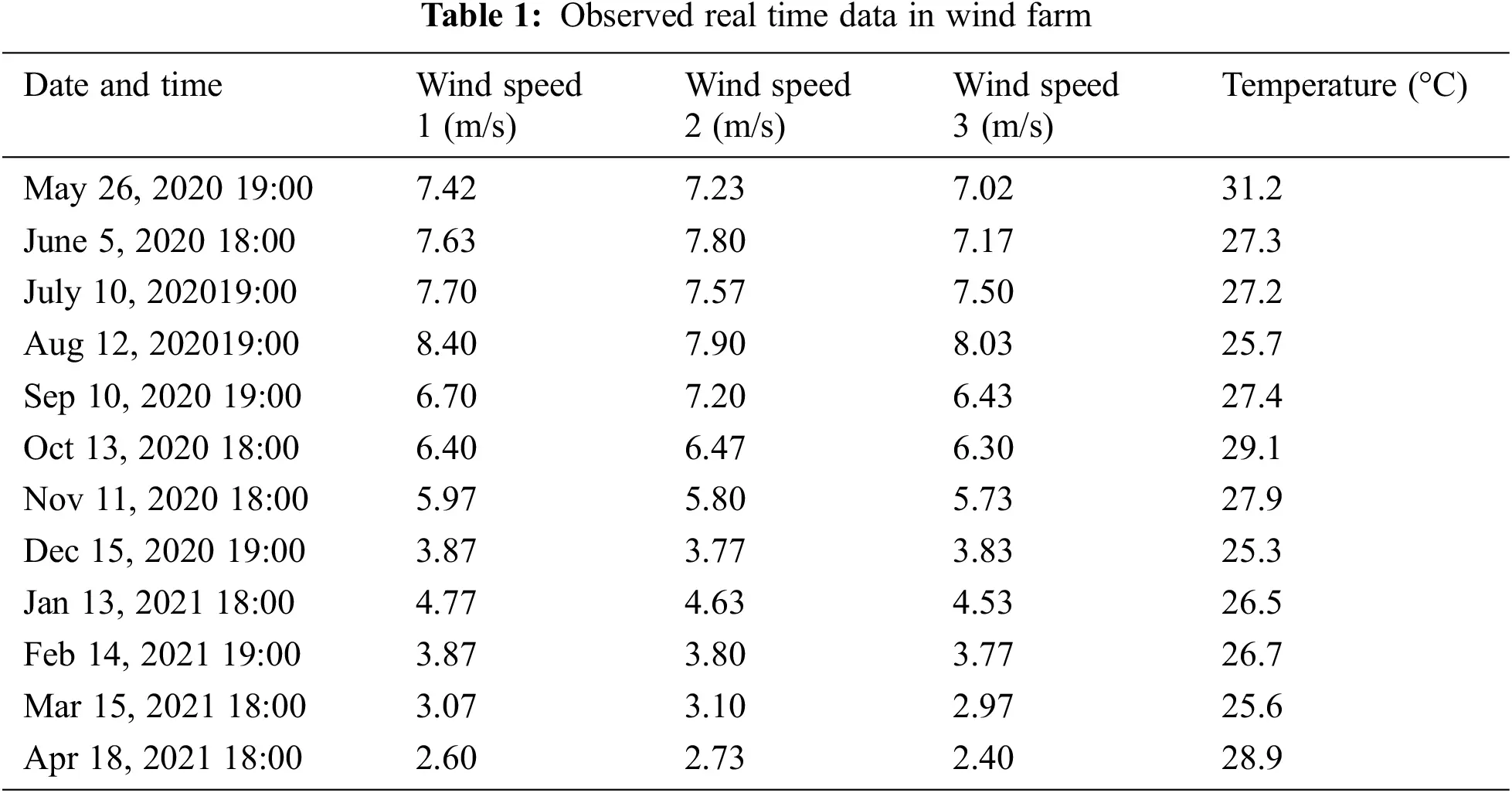The FLC is designed and simulated using the Matlab/Simulink block set. Fig. 3 shows the fuzzy logic controller implementation. Fuzzy rule viewer comprises of rules based on the IF-THEN logic. The inputs are combined using 49 fuzzy rules to produce the output. The following fuzzy sets are used for fuzzy reasoning. They are positive big (PB), positive medium (PM), positive small (PS), zero (Z), negative big (NB), negative medium (NM) and negative small (NS).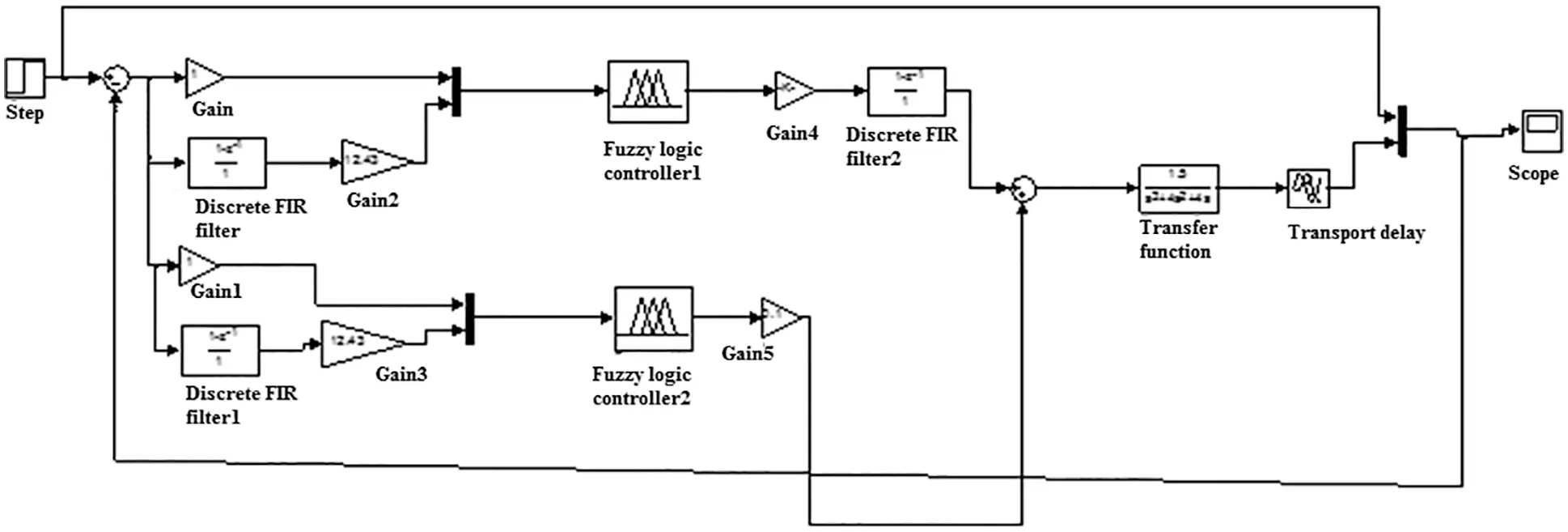Figure 3: Simulink model of fuzzy logic based frequency control

The fuzzy IF-THEN rules are utilized in FLC to perform frequency control which is provided in Tab. 2. Fuzzy logic based frequency control is carried out using frequency deviation (FD) and changes in wind speed (WS). Thus, these two parameters are considered as the input to FLC. Frequency stability needs to be maintained in the grid using frequency controller in the wind energy based hybrid systems. Since the frequency deviation indicates the changes observed in the standard frequency of 50 Hz, the observed changes in wind speed is utilized in the fuzzy reasoning. Fig. 4 shows the observed frequency variations from 20 June 2020 to 5 July 2020. The frequency variations are observed from 49.2 Hz to 50.6 Hz and it is important to maintain the frequency at 50 Hz.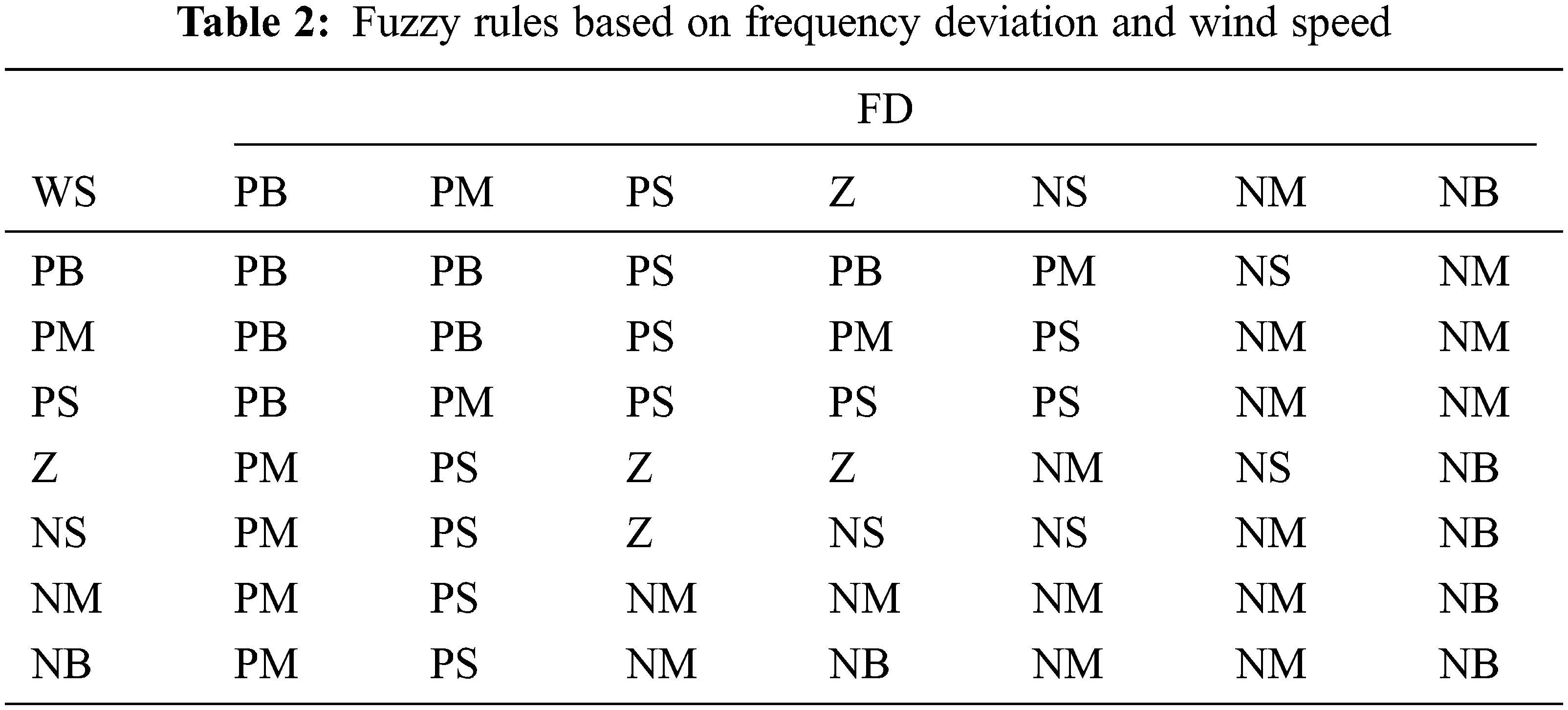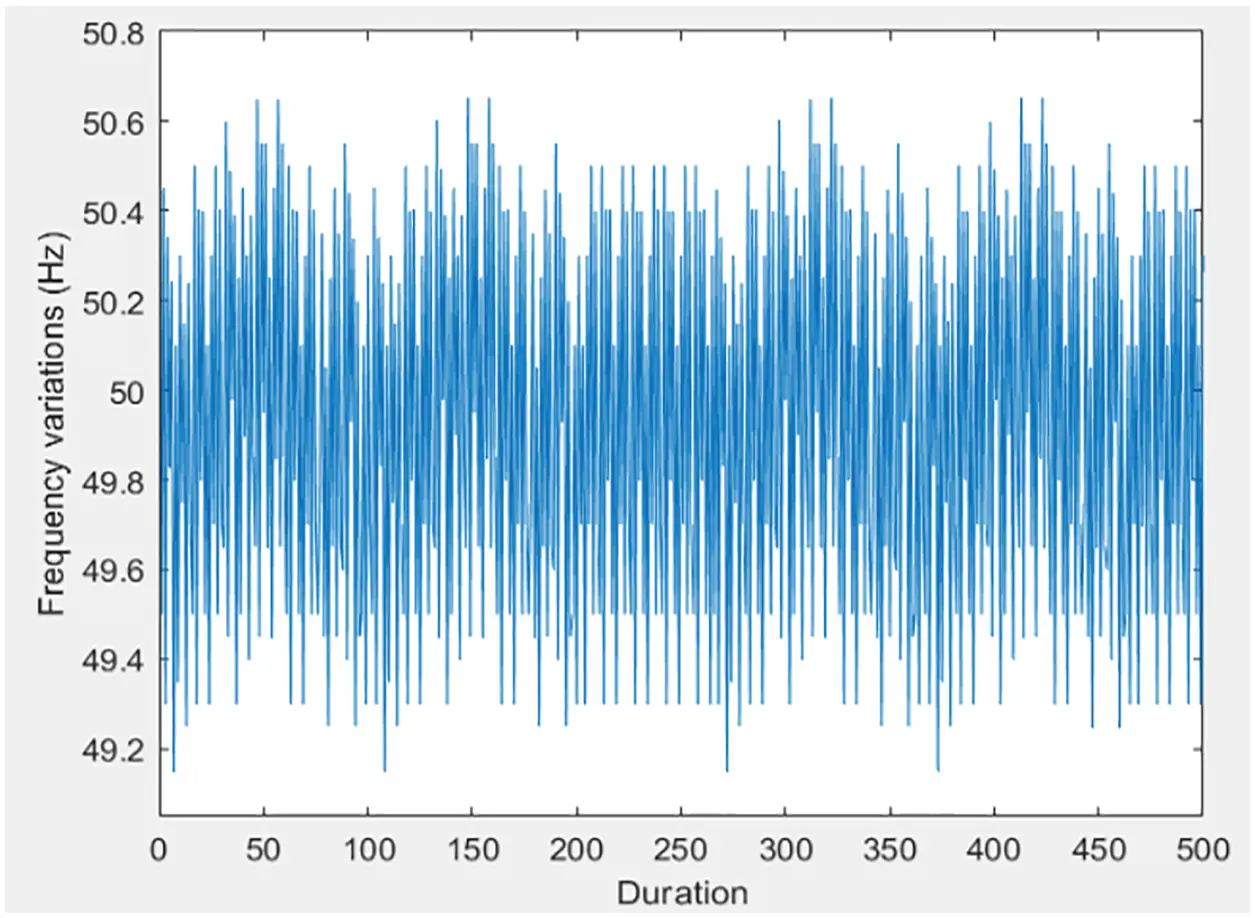Figure 4: Observed frequency variations

The wind speed in the selected wind farm location is usually high from May to October and very low during February to April. Wind speed variations are observed within a month or single day as well. Fig. 5 depicts the observed wind speed variations from 20 June to 5 July 2020.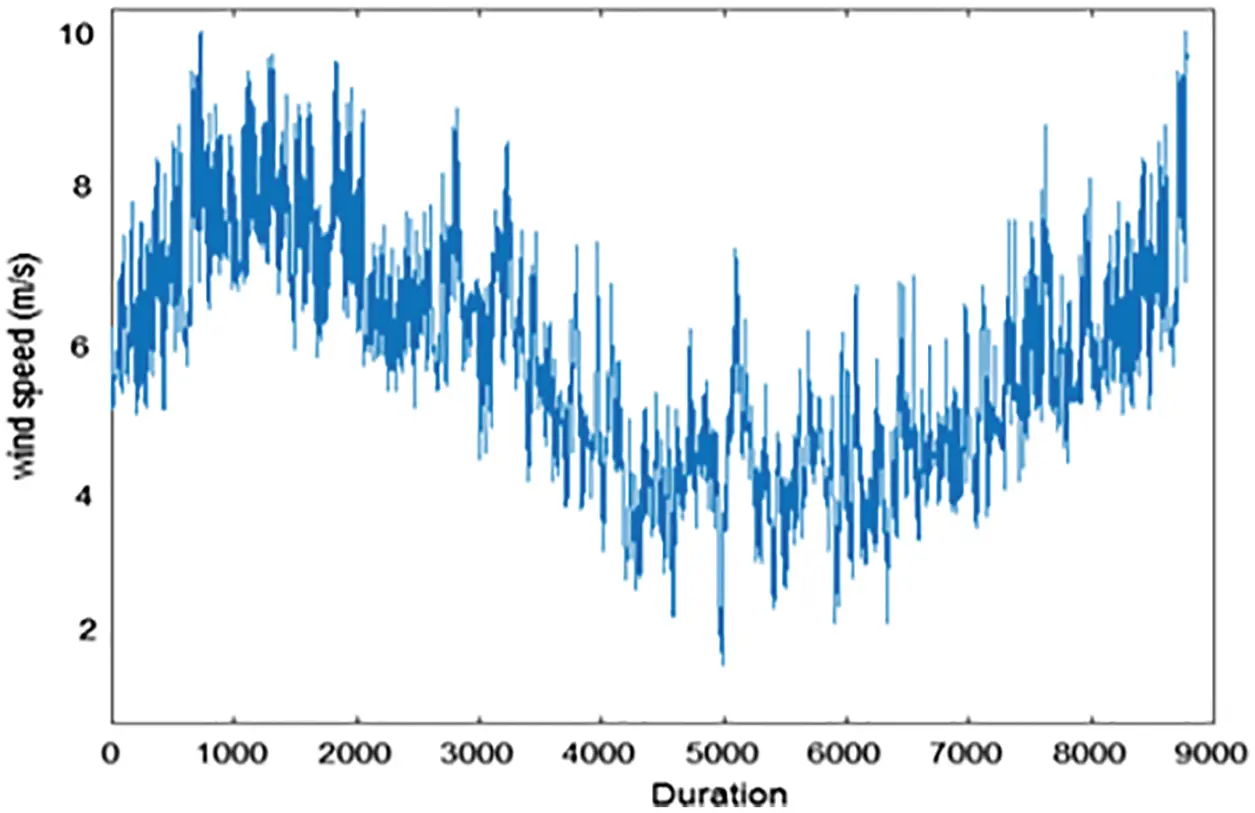Figure 5: Observed wind speed variations in collected data

The wind power generation cannot be predicted in most of the duration due to variable wind velocity situations. It is difficult to judge the required power adjustment based on the frequency deviation and variations in wind speed. As the variable inputs and required output have nonlinear relationship, fuzzy rules are developed based on the fuzzy reasoning provided in Tab. 2. The rule viewer of the proposed fuzzy logic based frequency control is depicted in Fig. 6. Fuzzy rules are written to relate the required power adjustment to the frequency deviation and variations in wind speed. It is required to provide more supply from wind generator whenever there is a decrease in frequency. However, it is not an easy task to calculate the required additional power due to variations in the wind speed. In view of these challenges, fuzzy logic controller is incorporated in this work.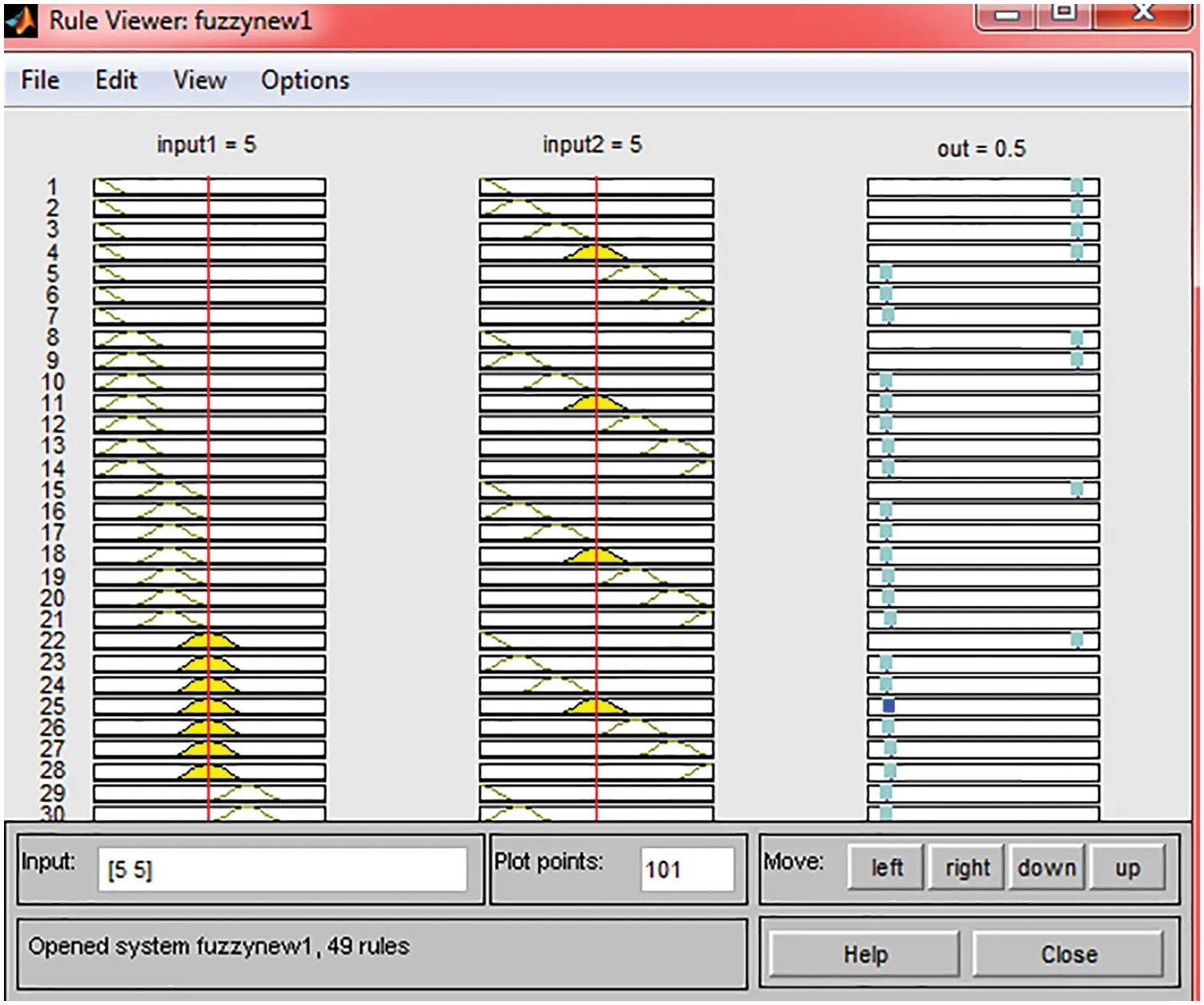Figure 6: Fuzzy rule viewer for frequency control

In this paper, MSE, RMSE, MAPE and NMAE are calculated to evaluate the performance of proposed frequency corrected demand forecasting. Fig. 7 compares the MAE of different approaches while measuring frequency corrected demand using one to nine minutes ahead forecasting. Kalman filter, ARMA model and various learning models are compared and the proposed Fuzzy+ANN+SVM is found to be better than all other approaches.Figure 7: Comparison of MAE in frequency corrected demand forecasting

Tab. 3 provides the MAPE values achieved from the proposed model on daily basis and weekly basis. All the results are compared with the existing AI based forecasting models. From Tab. 3, it is observed that MAPE is decreased by 41.1% than the existing wavelet and BPN based approach for May 2020 wind speed data.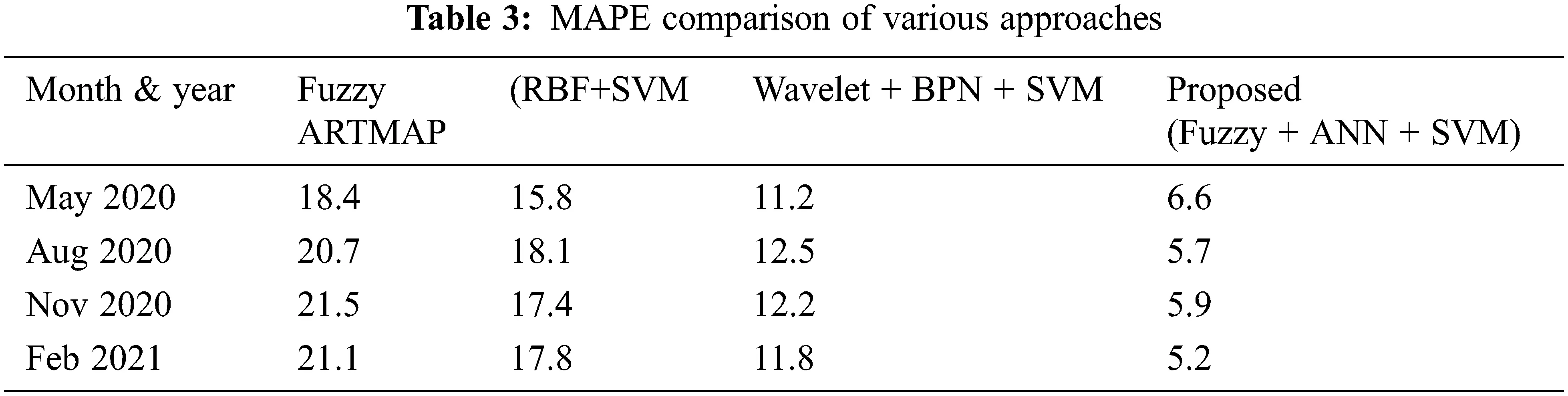The predicting performances of the models are found to be inconsistent with varying months. It is noticed that average of MAPEs of the four representative months found to have better comparison over all other models. The forecasting capability of the proposed hybrid model is superior to AI techniques and all the considered hybrid models. Tabs. 4 and 5 presents the RMSE and NMAE comparison of the proposed hybrid model with the existing models. In all cases, the proposed power system model outstrips the other model due to its capability to perform frequency correction. To improve the reliability and effectiveness of the developed model, simulations are approximately repeated 20-25 times for each case study. The test results show that the obtained NMAE values from the developed model are proven to be better than those obtained from the other models. Since the wind speed data contains various fluctuations, wavelets are used in decomposition and reconstruction to filter out those spikes. The RMSE and NMAE of the proposed forecasting is 6.12 and 3.76, which is the performance improvement of 9.9% and 23.1% respectively.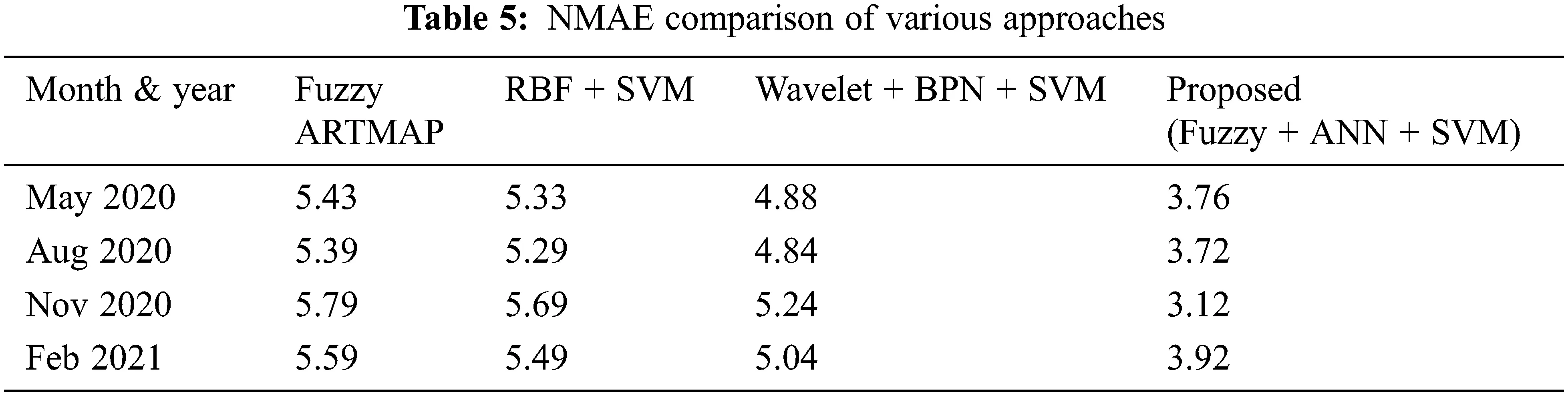From the above analysis, it is concluded that this control approach regulates the active power output of wind turbine according to the prediction information of wind and also maximizes usage of wind power.

5  Conclusion

The FLC based frequency correction technique is proposed for frequency corrected demand forecasting in wind based hybrid power systems. The MAE, MAPE, RMSE and NMAE are used to evaluate the performance of the proposed technique. It is worth mentioning that, the calculated MAE of proposed technique is found superior than all other existing forecasting methods. The MAPE is improved by 41.1% while comparing with the existing BPN and SVM based approaches. Similarly, RMSE and NMAE values are reduced by 9.9% and 23.1% respectively while comparing with other AI based models. Similarly, the implementation of FLC maintains frequency at constant value (50 Hz) and hence, the stability of the system is improved. In future, the prediction performance can be improved using probabilistic based models. The computational complexity analysis is needed to design an efficient prediction system. Hence, it is required to develop better forecasting system with computational efficiency.

Funding Statement: The authors received no specific funding for this study.

Conflicts of Interest: The authors declare that they have no conflicts of interest to report regarding the present study.

## References

1. M. Lange, “On the uncertainty of wind power predictions analysis of the forecast accuracy and statistical distribution of errors,” Journal of Solar Energy Engineering, vol. 127, no. 2, pp. 177–194, 2005.
2. Z. Xiaoliang, “Application of an improved droop control strategy in micro-grid,” International Journal of Frontiers in Engineering Technology, vol. 3, no. 3, pp. 44–49, 2021.
3. G. Giebel, C. Draxl, R. Brownsword, G. Kariniotakis and M. Denhard, The State-of-the-art in Short-term Prediction of Wind Power. A Literature Overview. Denmark, N. p., pp. 1–130, 2011.
4. P. Basak, S. Chowdhury, S. Halder Nee Dey and S. P. Chowdhury, “A literature review on integration of distributed energy resources in the perspective of control, protection and stability of micro grid,” Renewable and Sustainable Energy Reviews, vol. 16, no. 8, pp. 5545–5556, 2012.
5. Y. W. Zheng, M. Y. Chen, C. Li, R. Xu and X. Xu, “A micro grid control strategy based on adaptive drooping coefficient adjustment,” Automation of Electric Power Systems, vol. 37, no. 7, pp. 6–11, 2013.
6. H. Banakar, C. Luo and B. T. Ooi, “Impacts of wind power minute to minute variations on power system operation,” IEEE Transactions on Power Systems, vol. 23, no. 1, pp. 150–160, 2008.
7. H. Bevrani, F. Daneshfar and P. R. Daneshmand, “Intelligent power system frequency regulation concerning the integration of wind power units,” in Wind Power Systems. Berlin, Heidelberg: Springer, pp. 407–437, 2010.
8. H. Bevrani, A. Ghosh and G. Ledwich, “Renewable energy sources and frequency regulation: survey and new perspectives,” IET Renewable Power Generation, vol. 4, no. 5, pp. 438–457, 2010.
9. H. Bevrani and T. Hiyama, Intelligent Automatic Generation Control, 1st ed., vol. 1. Boca Raton, FL: CRC Press, 2011.
10. W. Bi, C. Chen and K. Zhang, “Optimal strategy of attack-defense interaction over load frequency control considering incomplete information,” IEEE Access, vol. 7, pp. 75342–75349, 2019.
11. S. Aziz, H. Wang, Y. Liu, J. Peng and H. Jiang, “Variable universe fuzzy logic-based hybrid LFC control with real-time implementation,” IEEE Access, vol. 7, pp. 25535–25546, 2019.
12. F. D. Gonzalez, M. Hau, A. Sumper and O. G. Bellmunt, “Participation of wind power plants in system frequency control: Review of grid code requirements and control methods,” Renewable and Sustainable Energy Reviews, vol. 34, pp. 551–564, 2014.
13. H. Zhao, Q. Wu, S. Hu, H. Xu and C. N. Rasmussen, “Review of energy storage system for wind power integration support,” Applied Energy, vol. 137, pp. 545–554, 2015.
14. M. Khalid and A. Savkin, “An optimal operation of wind energy storage system for frequency control based on model predictive control,” Renewable Energy, vol. 48, no. 3, pp. 127–132, 2012.
15. C. D. Das, A. K. Roy and N. Shinha, “GA based frequency controller for solar thermal diesel wind hybrid energy generation energy storage system,” International Journal of Electrical Power and Energy Systems, vol. 43, no. 1, pp. 262–279, 2012.
16. A. M. Howlader, Y. Izumi, A. Uehara, N. Urasaki, T. Senjyu et al., “A robust H controller based frequency control approach using the wind battery coordination strategy in a small power system,” International Journal of Electrical Power and Energy Systems, vol. 58, no. 1, pp. 190–198, 2014.
17. J. L. Torres, A. Garcia, M. De Blas and A. De Francisco, “Forecast of hourly average wind speed with ARMA models in Navarre (Spain),” Solar Energy, vol. 79, no. 1, pp. 65–77, 2005.
18. A. U. Haque and J. Meng, “Short-term wind speed forecasting based on fuzzy ARTMAP,” International Journal of Green Energy, vol. 8, no. 1, pp. 65–80, 2011.
19. M. Mohandes, T. Halawani, S. Rehman and A. A. Hussain, “Support vector machines for wind speed prediction,” Renewable Energy, vol. 29, no. 6, pp. 939–947, 2004.
20. J. Zhang, Y. M. Wei, D. Li, Z. Tan and J. Zhou, “Short term electricity load forecasting using a hybrid model,” Energy, vol. 158, no. 1, pp. 774–781, 2018.
21. Y. Wang, D. Gan, M. Sun, N. Zhang, Z. Lu et al., “Probabilistic individual load forecasting using pinball loss guided LSTM,” Applied Energy, vol. 235, pp. 10–20, 2019.
22. W. Sun, G. Dai, X. Zhang, X. He and X. Chen, “TBE-Net: A three-branch embedding network with part-aware ability and feature complementary learning for vehicle re-identification,” IEEE Transactions on Intelligent Transportation Systems, pp. 1–13, 2021.
23. W. Sun, L. Dai, X. R. Zhang, P. S. Chang and X. He, “RSOD: Real-time Small object detection algorithm in UAV-based traffic monitoring,” Applied Intelligence, vol. 92, no. 6, pp. 1–16, 2021.
24. K. Amarasinghe, D. L. Marino and M. Manic, “Deep neural networks for energy load forecasting,” in Proc. of the 2017 IEEE 26th Int. Symp. on Industrial Electronics (ISIE), Edinburgh, UK, pp. 1483–1488, 2017.
25. K. Chen, K. Chen, Q. Wang, Z. He, J. Hu et al., “Short-term load forecasting with deep residual networks,” IEEE Transactions on Smart Grid, vol. 10, no. 4, pp. 3943–3952, 2018.
26. C. Peng., Z. Zhang and J. Wu, “A wind power and load prediction based frequency control approach for wind-diesel-battery hybrid power system,” Mathematical Problems in Engineering, vol. 2015, pp. 1–7, 2015.
27. J. W. Taylor, “An evaluation of methods for very short-term load forecasting using minute-by-minute British data,” International Journal of Forecasting, vol. 24, no. 4, pp. 645–658, 2008.
28. S. Zhang, Y. Mishra and M. Shahidehpour, “Fuzzy-logic based frequency controller for wind farms augmented with energy storage systems,” IEEE Transactions on Power Systems, vol. 31, no. 2, pp. 1595–1603, 2016.This work is licensed under a Creative Commons Attribution 4.0 International License, which permits unrestricted use, distribution, and reproduction in any medium, provided the original work is properly cited.